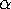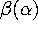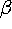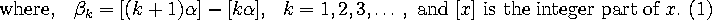# BERNOULLI (BETA) and INTEGER PART SEQUENCES

Rod Nillsen,Keith Tognetti and Graham Winley*
School of Mathematics and Applied Statistics,
University of Wollongong NSW 2522 Australia

A BERNOULLI or for brevity a Beta sequence for the real number, which we will represent byor simply, is defined as the infinite sequence :That isis simple the forward difference of the integer part sequence obtained from multiples of a real number.

Sequences of this type were first studied by Johann Bernoulli III (and hence the name Beta), the astronomer grandson of the famous mathematician Johann Bernoulli I. Although we are unable to identify the exact problems that stimulated his concern for these sequences, it probably had something to do with a cogwheel representation of planetary orbits which resulted in him being forced to calculate the integer parts of large multiples of irrationals. Without a computer this is very time consuming. However knowing the corresponding Beta sequence this becomes trivial using the property shown in P1 below.

Our interest in Beta sequences was aroused after reading some unpublished notes of Douglas Hofstadter , which gave a very spirited introduction to these sequences together with many fascinating examples; in particular they contain a description of the INT function which is rather cryptically described in his famous book "Gödel, Escher, Bach".

Johann III observed in 1772 , but did not prove, that in such sequences, having calculated the first few terms of the above integer parts formula (1), these terms can then be used to generate a larger number of terms and then this new subsequence can be used to generate an even larger subsequence and so on. Each time the increase in the number of terms is itself increasing which allows us to generate the sequence extremely rapidly. We describe a method for the rapid generation of the- sequence in detail in section 4 of this paper.